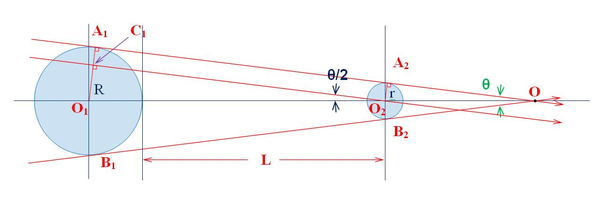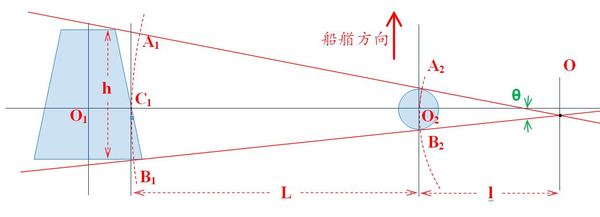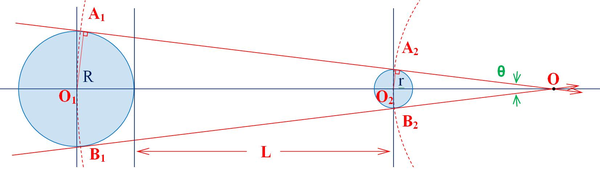ISTHMUS BUREAU OF SHIPPING DALIAN CO., LTD
 快速导航

# 关于失控灯水平遮蔽弧度计算的讨论

二维码

 英文原文如下：ANNEX I   POSITIONING AND TECHNICAL DETAILS OF LIGHTS AND SHAPES 9. Horizontal sectors (b) (i) All­round lights shall be so located as not to be obscured by masts, topmasts or structures within angular sectors of more than 6 degrees, except anchor lights prescribed in Rule 30, which need not be placed at an impracticable height above the hull. (ii) If it is impracticable to comply with paragraph (b)(i) of this section by exhibiting only one all­round light, two all­round lights shall be used suitably positioned or screened so that they appear, as far as practicable, as one light at a distance of one mile.O2圆心做A1A2的平行线，交O1A1C1。在三角形O1O2C1中，有下列等式成立：

(R r) = (R + L)*Sin (θ/2)

Sin (θ/2) = (R r) / (R + L)                        公式(1)

L = (R r) / Sin (θ/2) R                          公式(2)h = θ * (L + l) = θ*L + θ*l     (式中θ使用弧度rad)       公式(3)

2r = θ*l                    (式中θ使用弧度rad)       公式(4)

把式(4)代入式(3)中，我们得到式(5)

h = θ*L + 2r                (式中θ使用弧度rad)       公式(5)

θ = (h – 2r) / L   (弧度) = 180 * (h – 2r) / (π * L) (°)    （结论：大弧长减去小弧长然后除以两弧的间距等于两弧所对应的圆心角的弧度。）     公式(6)

L = (h 2r) / θ 用弧度) = 180 * (h 2r) / θ * π   °)       公式(7)

a) 对于圆柱形桅杆，可以利用公式(1)和公式(2)来精确计算；

b) 对任意形状的桅杆，包括圆柱形桅杆，都可以利用公式(6)和公式(7)来进行快速的近似计算；

环照灯半径r = d / 2 = 10 / 2 = 5 (厘米)

灯芯到主桅杆距离L = 1.5= 150 （厘米）

Sin (θ/2) = (R r) / (R + L) =11.14 5/11.14 + 150= 0.0381086

θ = 6°代入公式(2)，有：

L = (R r) / Sin (θ/2) R =11.14 5/Sin (6°/2) R= 106.19 （厘米）θ = (2R – 2r) / (R + L)   (弧度) = 180 * (2R – 2r) / (π * (R + L))   (°)

= 180*2* (11.14 5) / (11.14 + 150) / 3.14 = 4°.37

L + R = (2R 2r) / θ   用弧度) = 180 * (2R 2r) / θ * π   °)

L = 180 * (2R 2r) / (θ * π) R = 180 * 2 *(11.14 5) / 6 * 3.14 11.14

= 106.18 （厘米）

θ = h / L   (弧度) = 180 * h / (π * L) (°)                   公式(8)

θ = 180 * 2R / (π * (R + L)) = 180*2*11.14 / (3.14*(11.14 + 150)) = 7°.93

L = 180 * 2R / (π *θ) R = 180 * 2 * 11.14 / (3.14 * 6) 11.14 = 201.73 (cm)

L = 180 * 2R / (π *θ) = 180 * 2 * 11.14 / (3.14 * 6) = 212.87 (cm) = 2.13 ()

1） 两种计算方法的区别：

a) 方法一和方法二是以看到环照灯为准的计算方法；

b) 方法三是以看到环照灯的灯芯为准的计算方法。

2） 两种计算方法比较：

a) 当灯芯到大桅的距离一定时，以方法一和方法二计算的遮蔽角，以方法三来计算的遮蔽角将大于。同样，以方法三来计算的遮蔽角为时，以方法一和方法二计算的遮蔽角将小于

b) 当遮蔽角一定时，如遮蔽角为时，以方法一和方法二计算的灯芯到大桅桅杆的距离将小于以方法三来计算的距离。

3） 避碰公约里没有明确规定如何来确定遮蔽角，从上下文的意思理解看到灯光既可。因此，三种方法计算都可以。

4） 在实际应用中，出于支撑强度的考虑，支撑环照灯的横杆都尽可能取的比较短。此时采用方法一和方法二的计算，可以确定较短的灯芯到大桅的距离。